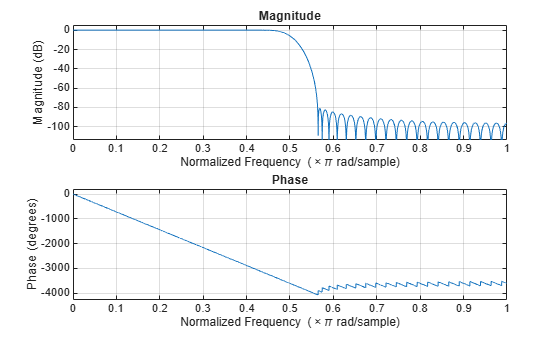# freqz

Frequency response of discrete-time filter System object

## Syntax

``````[h,w] = freqz(sysobj)``````
``````[h,w] = freqz(sysobj,n)``````
``````[h,w] = freqz(sysobj,'Arithmetic',arithType)``````
``freqz(sysobj)``

## Description

``````[h,w] = freqz(sysobj)``` returns the complex frequency response `h` of the filter System object™, `sysobj`. The vector `w` contains the frequencies (in radians/sample) at which the function evaluates the frequency response. The frequency response is evaluated at 8192 points equally spaced around the upper half of the unit circle.```
``````[h,w] = freqz(sysobj,n)``` returns the complex frequency response of the filter System object and the corresponding frequencies at `n` points equally spaced around the upper half of the unit circle.`freqz` uses the transfer function associated with the filter to calculate the frequency response of the filter with the current coefficient values.```
``````[h,w] = freqz(sysobj,'Arithmetic',arithType)``` analyzes the filter System object, based on the arithmetic specified in `arithType`, using either of the previous syntaxes.```

example

````freqz(sysobj)` uses `fvtool` to plot the magnitude and unwrapped phase of the frequency response of the filter System object `sysobj`.For more input options, see `freqz` in Signal Processing Toolbox™.```

## Examples

collapse all

This examples plot the frequency response of the lowpass FIR filter using `freqz`.

```b = fir1(80,0.5,kaiser(81,8)); firFilt = dsp.FIRFilter('Numerator',b); freqz(firFilt);```## Input Arguments

collapse all

Number of points over which the frequency response is computed. For an FIR filter where `n` is a power of two, the computation is done faster using FFTs.

Data Types: `single` | `double` | `int8` | `int16` | `int32` | `int64` | `uint8` | `uint16` | `uint32` | `uint64`

Arithmetic used in the filter analysis, specified as `'double'`, `'single'`, or `'Fixed'`. When the arithmetic input is not specified and the filter System object is unlocked, the analysis tool assumes a double-precision filter. When the arithmetic input is not specified and the System object is locked, the function performs the analysis based on the data type of the locked input.

The `'Fixed'` value applies to filter System objects with fixed-point properties only.

When the `'Arithmetic'` input argument is specified as `'Fixed'` and the filter object has the data type of the coefficients set to `'Same word length as input'`, the arithmetic analysis depends on whether the System object is unlocked or locked.

• unlocked –– The analysis object function cannot determine the coefficients data type. The function assumes that the coefficients data type is signed, has a 16-bit word length, and is auto scaled. The function performs fixed-point analysis based on this assumption.

• locked –– When the input data type is `'double'` or `'single'`, the analysis object function cannot determine the coefficients data type. The function assumes that the data type of the coefficients is signed, has a 16-bit word length, and is auto scaled. The function performs fixed-point analysis based on this assumption.

To check if the System object is locked or unlocked, use the `isLocked` function.

When the arithmetic input is specified as `'Fixed'` and the filter object has the data type of the coefficients set to a custom numeric type, the object function performs fixed-point analysis based on the custom numeric data type.

## Output Arguments

collapse all

Complex `n`-element frequency response vector. If `n` is not specified, the function uses a default value of 8192. The frequency response is evaluated at `n` points equally spaced around the upper half of the unit circle.

Data Types: `double`
Complex Number Support: Yes

Frequency vector of length `n`, in radians/sample. `w` consists of `n` points equally spaced around the upper half of the unit circle (from 0 to π radians/sample). If `n` is not specified, the function uses a default value of 8192.

Data Types: `double`

## Tips

There are several ways of analyzing the frequency response of filters. `freqz` accounts for quantization effects in the filter coefficients, but does not account for quantization effects in filtering arithmetic. To account for the quantization effects in filtering arithmetic, refer to function `noisepsd`.

## Algorithms

`freqz` calculates the frequency response for a filter from the filter transfer function Hq(z). The complex-valued frequency response is calculated by evaluating Hq(ejω) at discrete values of w specified by the syntax you use. The integer input argument `n` determines the number of equally-spaced points around the upper half of the unit circle at which `freqz` evaluates the frequency response. The frequency ranges from 0 to π radians per sample when you do not supply a sampling frequency as an input argument. When you supply the scalar sampling frequency `fs` as an input argument to `freqz`, the frequency ranges from 0 to `fs`/2 Hz.

## Version History

Introduced in R2011a